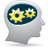## Negative float value print

cancel
Showing results for
Search instead for
Did you mean:

## Negative float value print

398 ViewsContributor V

Hi in KDS 3.2 and latest SDK for K64F, this code:

double var1 = -5.8;

PRINTF("%f\r\n", var1);

double var2 = -10.4;

PRINTF("%f\r\n, var2);

double var3 = 1.5;

PRINTF("%f\r\n", var3);

produces:

-6.8

-11.4

1.5

i.e. negative double numbers are printed with -1 added to them. Why and how to fix it?

Labels (1)
• ### General

3 Replies
175 ViewsNXP Employee

Hi Martin,

There is bug in the fsl_debug_console.c (look below for "//DES" comment).

static int32_t DbgConsole_ConvertFloatRadixNumToString(char *numstr,
void *nump,
int32_t radix,
uint32_t precision_width)
{
int32_t a;
int32_t b;
int32_t c;
int32_t i;
uint32_t uc;
double fa;
double dc;
double fb;
double r;
double fractpart;
double intpart;

int32_t nlen;
char *nstrp;
nlen = 0;
nstrp = numstr;
*nstrp++ = '\0';
r = *(double *)nump;
if (!r)
{
*nstrp = '0';
++nlen;
return nlen;
}
fractpart = modf((double)r, (double *)&intpart);
/* Process fractional part. */
for (i = 0; i < precision_width; i++)
{
fractpart *= radix;
}
if (r >= 0)
{
fa = fractpart + (double)0.5;
if (fa >= pow(10, precision_width))
{
fractpart++; //DES was intpart++;
}
}
else
{
fa = fractpart - (double)0.5; //DES???
if (fa <= pow(-10, precision_width))
{
fractpart--; //DES was intpart--;
}
}

Please see solution on this post: PRINTF in KDS

Regards,

David

175 ViewsContributor III

Interestingly, I applied this fix last summer in order to fix the off-by-one error when printing negative floats.  However, I've just noticed another corner case:

PRINTF("%4.1f\r\n", 7.94); // ----- displays "7.9" as you'd expect
PRINTF("%4.1f\r\n", 7.96); // ----- displays "7.0" instead of 8.0

175 ViewsContributor IV

Hello

we are using "%ld" for same application

so

can you try with "%ld " rather than "%f"